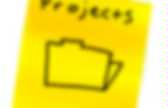# Kalman Filter

Digital Filter Design. The basic idea behind the Window method of filter design is that the ideal frequency response of the desired filter is equal to 1 for all the pass band frequencies, and equal to 0 for all the stop band frequencies, then the filter impulse response is obtained by taking the Discrete Fourier Transform (DFT) of the ideal frequency response.Unfortunately, the filter response would be infinitely long since it has to reproduce the infinitely steep discontinuities in the ideal frequency response at the band edges. To create a Finite Impulse Response (FIR) filter, the time domain filter coefficients must be restricted in number by multiplying by a window function of a finite width. The simplest window function is the rectangular window which corresponds to truncating the sequence after a certain number of terms. Kaiser-Bessel filter generator FIR Listing Kaiser-Bessel filter design formulae The methods used in this FIR generator were taken from the paper by J.

M \ge \frac{Att - 8}{14.36\ dF/{Fs}} Kalman filter. The Kalman filter keeps track of the estimated state of the system and the variance or uncertainty of the estimate.The estimate is updated using a state transition model and measurements. denotes the estimate of the system's state at time step k before the k-th measurement yk has been taken into account; is the corresponding uncertainty. The algorithm works in a two-step process. In the prediction step, the Kalman filter produces estimates of the current state variables, along with their uncertainties. It is a common misconception that the Kalman filter assumes that all error terms and measurements are Gaussian distributed. Extensions and generalizations to the method have also been developed, such as the extended Kalman filter and the unscented Kalman filter which work on nonlinear systems.

Naming and historical development The filter is named after Hungarian émigré Rudolf E. Kalman filters have been vital in the implementation of the navigation systems of U.S. Where Details Cookbook/KalmanFiltering - This is code implements the example given in pages 11-15 of An Introduction to the Kalman Filter by Greg Welch and Gary Bishop, University of North Carolina at Chapel Hill, Department of Computer Science.It produces plots that look something like this: Estimate vs. iteration step Estimated a priori error vs. iteration step CategoryCookbook. Pykalman — pykalman 0.9.2 documentation. Kalman Filters for Undergrads Part I: Linear Kalman Filters. Introduction Kalman filters let you use mathematical models despite having error-filled real-time measurements.Programmers dealing with real-world data should know them. Publications explaining Kalman filters are hard for Computer Scientists/Engineers to understand since they expect you to know control theory. I wrote this guide for people who want to learn Kalman filters but never took a control theory course. This guide will cover : When Kalman filters can help. Examples of solving simple problems with Kalman filters. Examples of how to convert normal-looking equations into Kalman filter matrices.

Example code implementing Kalman filters in Python. This guide WON'T cover : Kalman filter history. When and why Kalman filters are optimal. How to tune Kalman filters for performance. In-depth details (such as exceptions to guidelines).